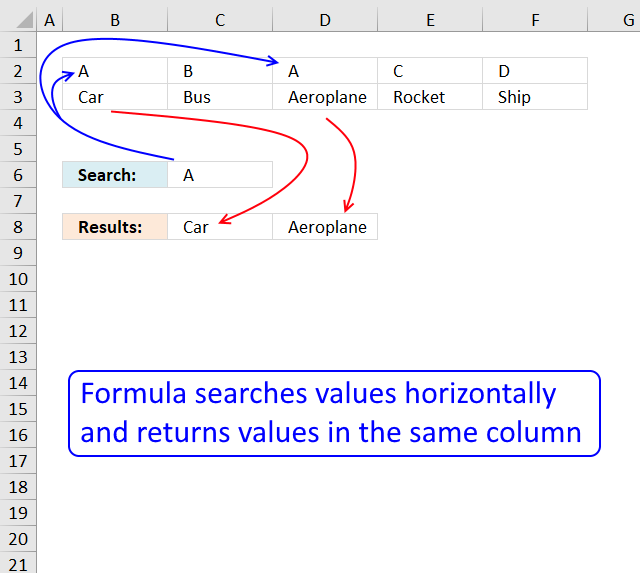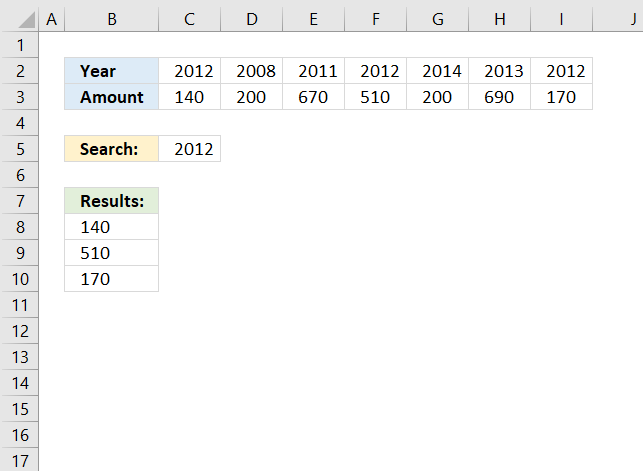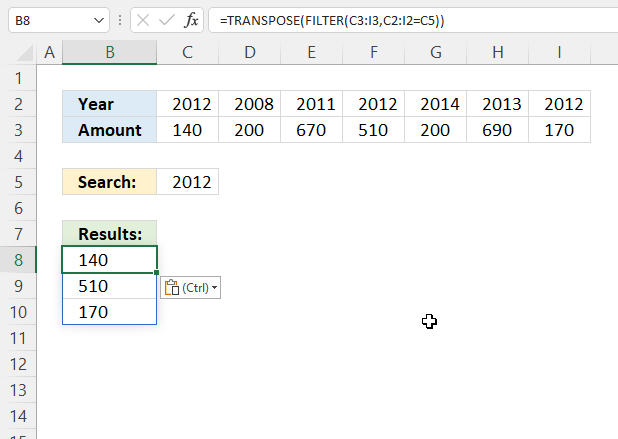Author: Oscar Cronquist Article last updated on August 26, 2022## 1. Search values distributed horizontally and return the corresponding value

Question: Hi, The formula here works great but I can't figure out how to change it to work with data in columns.

Here is what I have:

=INDEX(A2:E2,SMALL(IF(A1:E1=A3,COLUMN(A1:E1),""),COLUMN()))

A B C D E
1 A B A C D
2 Car Bus Aeroplane Rocket Ship
3 A

I'd expect the result to read:

A B
4 Car Aeroplane

A B
4 #NUM #NUM

This is a question from Using array formula to look up multiple values in a listArray formula in cell B8:

=INDEX(\$C\$3:\$I\$3, SMALL(IF(\$C\$2:\$I\$2=\$C\$5, MATCH(COLUMN(\$C\$2:\$I\$2), COLUMN(\$C\$2:\$I\$2)), ""), ROWS(\$A\$1:A1)))

To enter an array formula, type the formula in a cell then press and hold CTRL + SHIFT simultaneously, now press Enter once. Release all keys.

The formula bar now shows the formula with a beginning and ending curly bracket telling you that you entered the formula successfully. Don't enter the curly brackets yourself.

### Explaining formula in cell B8

#### Step 1 - Check if lookup value is equal to values in cell range C2:I2

The IF function has three arguments, the first one must be a logical expression. If the expression evaluates to TRUE then one thing happens (argument 2) and if FALSE another thing happens (argument 3). The following lines explain the logical expression:

\$C\$2:\$I\$2=\$C\$5

becomes

{2012,2008,2011,2012,2014,2013,2012}=2012

and returns

{TRUE,FALSE,FALSE,TRUE,FALSE,FALSE,TRUE}

#### Step 2 - Return corresponding column number

The column number will help us identify the values we want to return from another row. TRUE - corresponding column number, FALSE - nothing "".

IF(\$C\$2:\$I\$2=\$C\$5, MATCH(COLUMN(\$C\$2:\$I\$2), COLUMN(\$C\$2:\$I\$2)), "")

becomes

IF({TRUE,FALSE,FALSE,TRUE,FALSE,FALSE,TRUE}, MATCH(COLUMN(\$C\$2:\$I\$2), COLUMN(\$C\$2:\$I\$2)), "")

becomes

IF({TRUE,FALSE,FALSE,TRUE,FALSE,FALSE,TRUE}, {1,2,3,4,5,6,7}, "")

and returns

{1,"","",4,"","",7}.

#### Step 3 - Extract k-th smallest column number

To be able to return a new value in a cell each I use the SMALL function to filter column numbers from smallest to largest.

The ROWS function keeps track of the numbers based on an expanding cell reference. It will expand as the formula is copied to the cells below.

SMALL(IF(\$C\$2:\$I\$2=\$C\$5, MATCH(COLUMN(\$C\$2:\$I\$2), COLUMN(\$C\$2:\$I\$2)), ""), ROWS(\$A\$1:A1))

becomes

SMALL({1,"","",4,"","",7}, ROWS(\$A\$1:A1))

becomes

SMALL({1,"","",4,"","",7}, 1)

and returns 1.

#### Step 4 - Return value based on column number

The INDEX function returns a value based on a cell reference and column/row numbers.

INDEX(\$C\$3:\$I\$3, SMALL(IF(\$C\$2:\$I\$2=\$C\$5, MATCH(COLUMN(\$C\$2:\$I\$2), COLUMN(\$C\$2:\$I\$2)), ""), ROWS(\$A\$1:A1)))

becomes

INDEX(\$C\$3:\$I\$3, 1)

becomes

INDEX({140,200,670,510,200,690,170}, 1)

and returns 140 in cell B8.

### Get the Excel fileLookup-horizontally-and-return-multiple-values.xlsx

## 2. Filter values distributed horizontally - Excel 365The FILTER function is capable to filter values arranged horizontally as well, the TRANSPOSE function rearranges the result vertically.

Excel 365 formula in cell B8:

=TRANSPOSE(FILTER(C3:I3,C2:I2=C5))

### Explaining formula

#### Step 1 - Logical test

The equal sign is a logical operator, it allows you to compare value to value. The result is a boolean value TRUE or FALSE.

C2:I2=C5

becomes

{2012,2008,2011,2012,2014,2013,2012}=2012

and returns

{TRUE, FALSE, FALSE, TRUE, FALSE, FALSE, TRUE}.

#### Step 2 - Filter values

The FILTER function extracts values/rows based on a condition or criteria.

Function syntax: FILTER(array, include, [if_empty])

FILTER(C3:I3,C2:I2=C5)

becomes

FILTER({140, 200, 670, 510, 200, 690, 170},{TRUE, FALSE, FALSE, TRUE, FALSE, FALSE, TRUE})

and returns

{140, 510, 170}.

#### Step 3 - Transpose values

The TRANSPOSE function converts a vertical range to a horizontal range, or vice versa.

Function syntax: TRANSPOSE(array)

TRANSPOSE(FILTER(C3:I3,C2:I2=C5))

becomes

TRANSPOSE({140, 510, 170})

and returns

{140; 510; 170}.